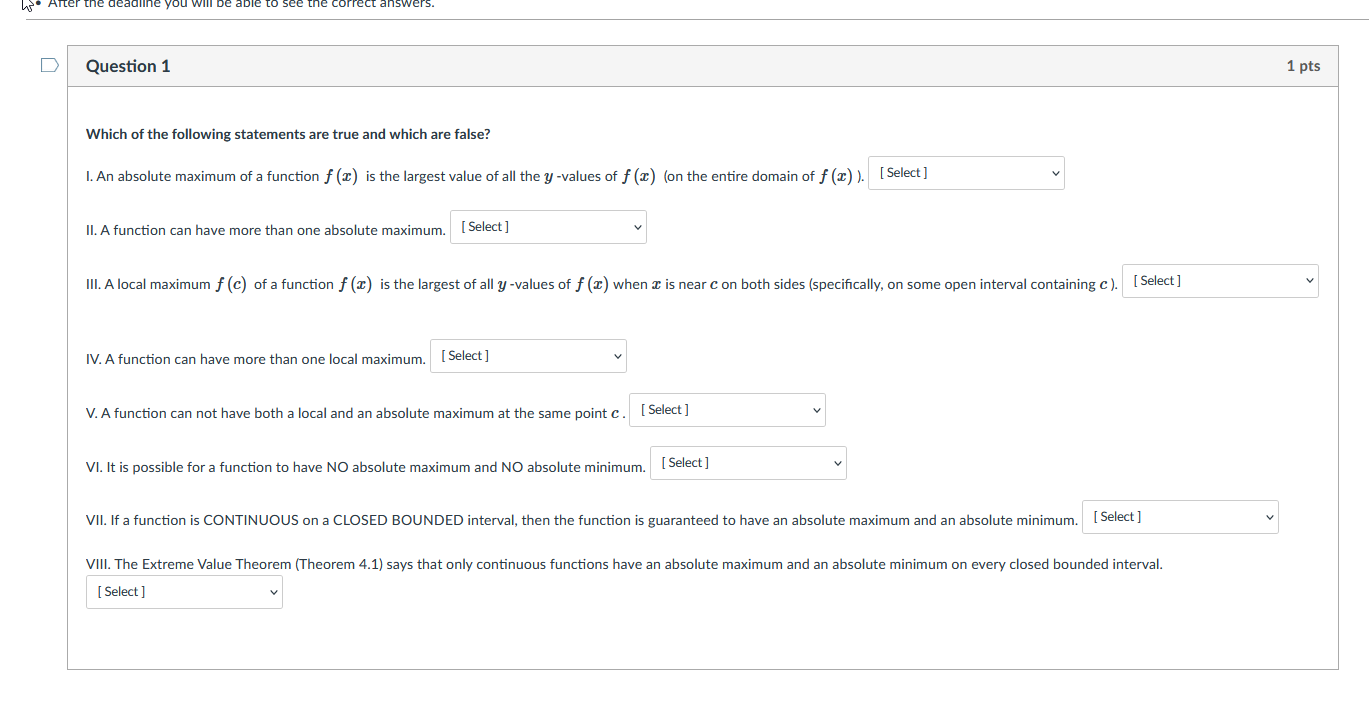# Question W Alter the deadline you will be able the correct answers. Question 1 1 pts Which of the following statements are true and which are false? 1. An absolute maximum of a function f (2) is the largest value of all the y-values of f(T) (on the entire domain of f(c)). [Select] II. A function can have more than one absolute maximum. [Select] II. A local maximum f (c) of a function f (2) is the largest of all y-values of f (2) when I is near con both sides (specifically, on some open interval containing c). [Select] IV. A function can have more than one local maximum. [ Select] V. A function can not have both a local and an absolute maximum at the same point c. Select ] VI. It is possible for a function to have NO absolute maximum and NO absolute minimum. VII. If a function is CONTINUOUS on a CLOSED BOUNDED interval, then the function is guaranteed to have an absolute maximum and an absolute minimum. (Select] VIII. The Extreme Value Theorem (Theorem 4.1) says that only continuous functions have an absolute maximum and an absolute minimum on every closed bounded interval. [ Select ]